# Reflect the value changed in the action after post of the form in the view

Page creation date :

## environment

Visual Studio
• Visual Studio 2022
ASP.NET Core
• 6.0 MVC

It works in environments other than the above, but the published code was written in the framework above.

## What to make

Try to create a simple program that says, "Post the text you typed on the screen and return the processed text to the same screen as a new result."

## code

The base is the creation of a new MVC project, from which you add code. The code is published for the overall configuration, so please refer to it.

### Model (viewmodel)

Create the following model for view and action interaction: `ResultValue` There are two, but I wanted to give two results in the view, so I prepared two.

SampleViewModel

``````namespace PostValueChange.Models
{
public class SampleViewModel
{
/// <summary>入力値を受け取るプロパティ。</summary>
public string? InputValue { get; set; }

/// <summary>結果を出力するプロパティ。</summary>
public string? ResultValue1 { get; set; }

/// <summary>結果を出力するプロパティ。</summary>
public string? ResultValue2 { get; set; }
}
}
``````

### action

It's a simple process: get to display the view, take the text entered in POST, process it, and return the result.

HomeController

``````// 省略

namespace PostValueChange.Controllers
{
public class HomeController : Controller
{
// 省略

[HttpGet]
public IActionResult Sample() => View();

[HttpPost]
public IActionResult Sample(SampleViewModel model)
{
if (ModelState.IsValid == false) View(model);
model.ResultValue1 = model.InputValue + " テキストを追加1";
model.ResultValue2 = model.InputValue + " テキストを追加2";
return View(model);
}
}
}
``````

### view

Create views based on actions and models.

When you `ResultValue1` click the update button, and `ResultValue2` are displayed, but they are displayed in "input text" and "in div tag", respectively.

Sample.cshtml

``````@model PostValueChange.Models.SampleViewModel

@{
ViewData["Title"] = "Sample";
}

<h1>Sample</h1>

<h4>SampleViewModel</h4>
<hr />
<div class="row">
<div class="col-md-4">
<form asp-action="Sample" >
<div asp-validation-summary="ModelOnly" class="text-danger"></div>
<div class="form-group">
<label asp-for="InputValue" class="control-label"></label>
<input asp-for="InputValue" class="form-control" />
<span asp-validation-for="InputValue" class="text-danger"></span>
</div>
<div class="form-group">
<input type="submit" value="更新" class="btn btn-primary" />
</div>
<div class="form-group">
<label asp-for="ResultValue1" class="control-label"></label>
<input asp-for="ResultValue1" class="form-control" />
<span asp-validation-for="ResultValue1" class="text-danger"></span>
</div>
<div>@Model?.ResultValue2</div>
</form>
</div>
</div>

<div>
<a asp-action="Index">前の画面に戻る</a>
</div>

@section Scripts {
@{await Html.RenderPartialAsync("_ValidationScriptsPartial");}
}
``````

`Index.cshtml` Add a link so that you can transition from `Sample` to .

Index.cshtml

``````@{
}

<div class="text-center">
<h1 class="display-4">Welcome</h1>
<p>Learn about <a href="https://docs.microsoft.com/aspnet/core">building Web apps with ASP.NET Core</a>.</p>
</div>

<a asp-action="Sample">Sample</a>    @* 追加 *@
``````

## Updating the model received in the POST process does not affect the input of the view, etc.

Run the code you created above, enter from `InputValue` the Sample screen, and try to update.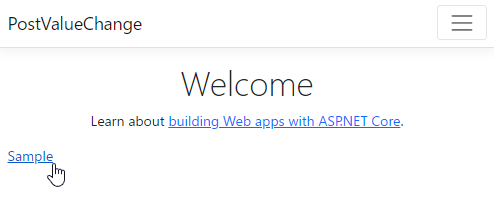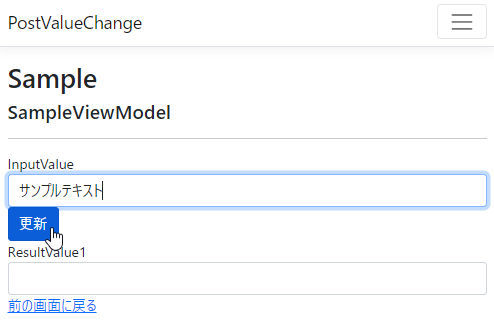On the action side, `ResultValue1` since the values are set to and `ResultValue2` returned, it is assumed that both are originally displayed, but in fact `div` only the 2 set in the tag are displayed.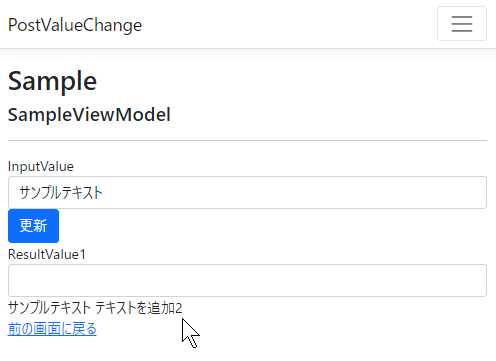### The value set to ModelState takes precedence

When the client POSTs the input value, the value is set to the model variable of the `ControllerBase.ModelState` argument, but the value is also set. The value entered is `ModelState` then validated with the value set to . `ModelState.IsValid` That is why it is judged in. When debugging, you can check the contents by stopping processing at the breakpoint.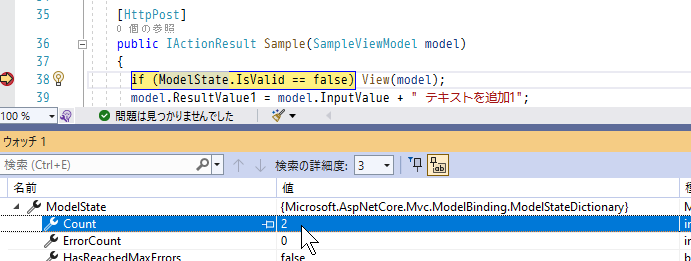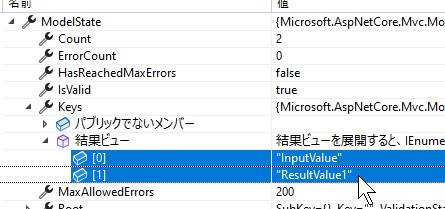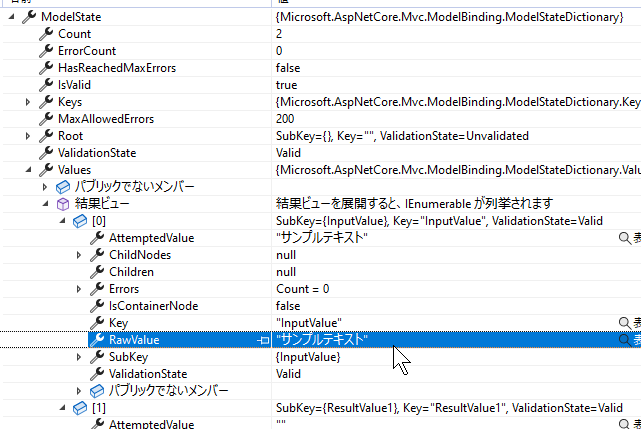If you originally want to return a value to the view, it is `return View(model);` OK to include , but if a value is `ModelState` set to ,`ModelState` the value of takes precedence and is returned to the view.

`ModelState` Since the value set to is a value sent by inputting the view, etc., in terms of `InputValue`the model, , `ResultValue1` is set. Therefore,`ResultValue1` the value of takes `ModelState` precedence,`ModelState` and the value set in the model that is not `ResultValue2` set to is displayed in the view.

## To return a value to a view in favor of a model value

`ModelState` Values set to take precedence to the view, so if `ModelState` you erase the values set to , the value of the model will be returned to the view.

`ModelState.Clear()` You can erase all the values that has by `ModelState` calling as follows:

``````[HttpPost]
public IActionResult Sample(SampleViewModel model)
{
if (ModelState.IsValid == false) View(model);

// ModelState の値を消してモデルの値をビューに返却できるようにする
ModelState.Clear();

// ビューに返す値を設定する
model.ResultValue1 = model.InputValue + " テキストを追加1";
model.ResultValue2 = model.InputValue + " テキストを追加2";

return View(model);
}
``````

`ModelState` is also doing the validation process,`ModelState.Clear` so be sure `ModelState.IsValid` to read it after when you call .

When you run it, the result will be displayed correctly as follows.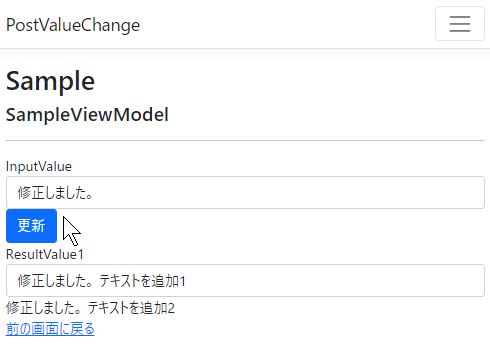`ModelState.Clear` Since all values disappear when you call a method, it is also possible to specify the property name of the model and erase the values individually as follows.

``````[HttpPost]
public IActionResult Sample(SampleViewModel model)
{
if (ModelState.IsValid == false) View(model);

// ModelState の値を消してモデルの値をビューに返却できるようにする
ModelState.Remove(nameof(model.ResultValue1));

// ビューに返す値を設定する
model.ResultValue1 = model.InputValue + " テキストを追加1";
model.ResultValue2 = model.InputValue + " テキストを追加2";

return View(model);
}
``````